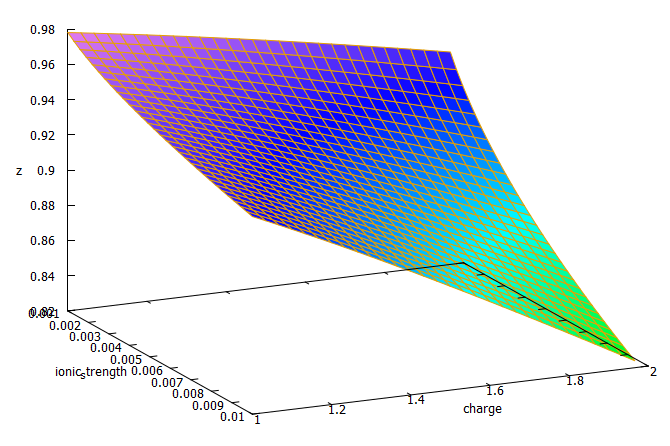# Improving on HydroBuddy’s theoretical conductivity model, the LMCv2Hydrobuddy’s theoretical conductivity estimates have never been good. As I discussed in a previous post, the program uses a very simple model based on limiting molar conductivities to calculate the EC. The software knows how much each ion conducts when it’s all by itself, so it adds all these conductivity values multiplied by the concentration and assumes there are no additional effects. The conductivity values resulting from this assumption are very large – because there are effects that significantly reduce the conductivity of ions at larger concentrations – so HydroBuddy just cuts the estimation by 35% hoping to reach more accurate values. This works great for some cases, but very badly for others.

The reason why this happens is that the actual conductivity contribution of some ions decreases more drastically as a function of concentration and due to the presence of other ions compared to others. This means that we need to account for these decreases in conductivity in an ion-specific way. One way to approach this, is to forget about theoretical approximations and just create an empirical model that uses experimental data. This is what I did when I created the empirical model that is present in HydroBuddy from v1.7. This model works really well, provided you are using the exact list of salts that were used to create the model and you stay within the boundaries of concentration values that were used to create it.Equations 1-3 were taken from here. I have then used these equations to derive equation 4, which is going to be the new LMCv2 model for HydroBuddy from v1.9. Where Λ0m,i is the limiting molar conductivity of each ion, zi is each ion’s charge, I is the ionic strength of the solution and ci is the molar concentration of each ion..

This experiment-based solution can be great. It is in fact, a technique I’ve used to create custom versions of HydroBuddy for clients who want to have high accuracy in their EC estimations within the salts that they specifically use. The process is however cumbersome and expensive, my wife and I – both of us chemists – do all the experimentation, and it generally requires an entire day, preparing more than 80+ solutions using high accuracy volumetric material, to get all the experimental data. It is also limited in scope, as any salt change usually requires the preparation of a substantial number of additional solutions to take it into consideration.

It would certainly be great if we could create a better, fully theoretical, conductivity model. Diving into the literature and programs used for conductivity-related calculations, I found a program called Aqion that implements a more accurate model compared with HydroBuddy’s LMC model. You can read more about their approach here. They use the limiting molar conductivities but introduce additional terms to make ion-specific corrections that are related to both ionic charge and ionic strength. The ionic charge is the electrical charge of each ion, for example, +1 for K+ and +2 for Fe+2, etc. The ionic strength is the sum of the molar concentration of each ion times its charge.3D plot of equation 4 showing the magnitude of the correction factor (z) as a function of charge and ionic strength.

The plot above shows you how this correction factor affects a solution as the ionic strength and charge of the ions change. As a solution gets more diluted, the equation approaches the sum of the conductivities at infinite dilution. Conversely, as the solution becomes more concentrated or the ion charge becomes higher, the drop in the conductivity becomes more pronounced. These are both phenomena that are in-line with experimental observations and much better reflect how conductivity is supposed to change when different ions interact in solution.

The above equation provides us with a more satisfactory theoretical estimation of conductivity compared to the current HydroBuddy LMC model. The new model is able to implement correction factors on a per-ion basis and also changes the magnitude of these corrections depending on how concentrated the solutions are. This new model will be implemented to replace the current LMC model in HydroBuddy v1.9, which will be released in the near future. This should provide significantly more accurate estimates of conductivity for the preparation of hydroponic solutions.

Subscribe Today!